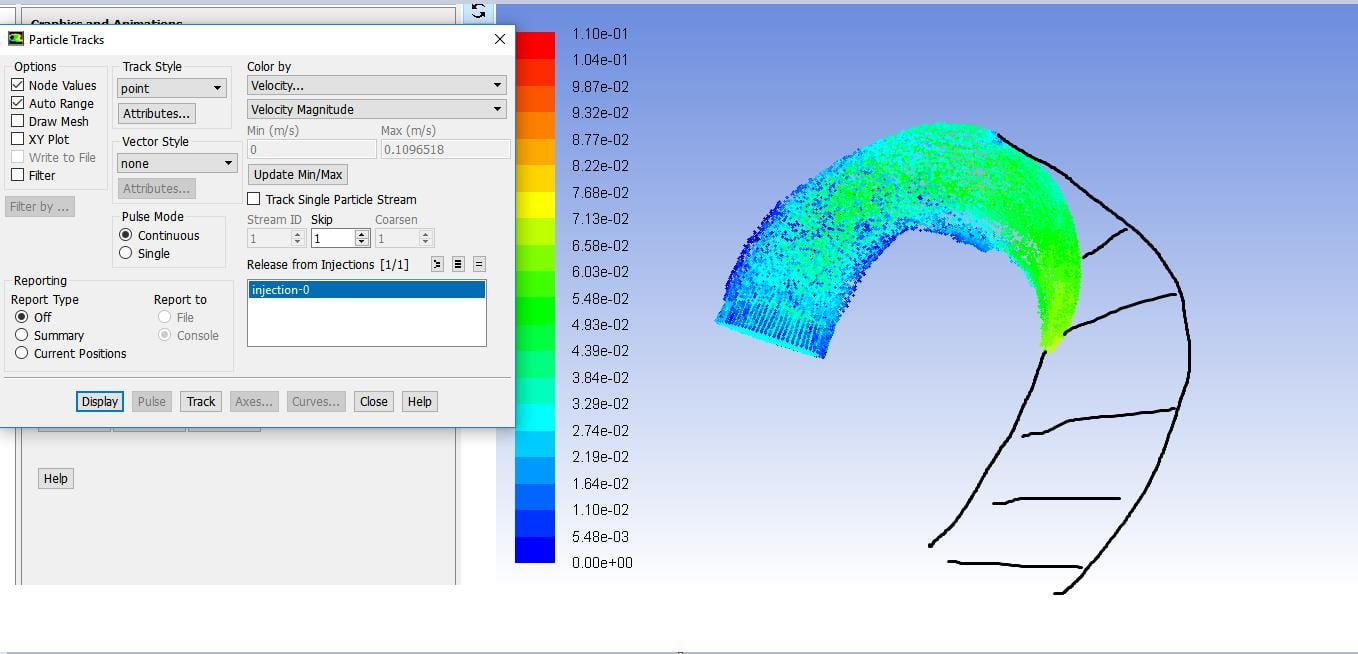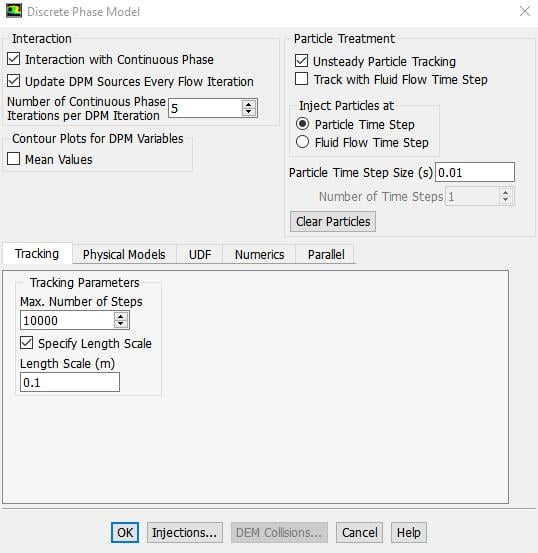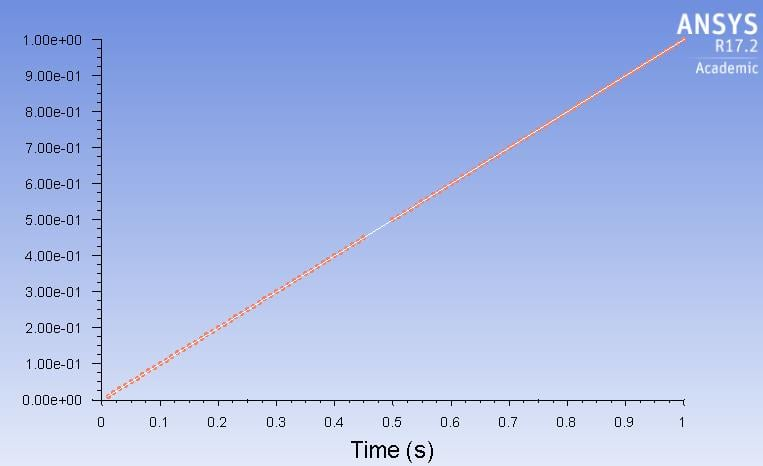## Fluids

•Kamukyo
Subscriber

Hello,

I used discrete phase model (DPM) to track the particles.

But, it seemed to be incomplete since the particle should be distributed over the entire area (the particle in the area highlighted with the black is missing).

I set Max number of steps = 5000000, step length factor = 5 with unsteady particle tracking option.

Injection type is the surface (Inlet) and the particle type is the massless.

number tracked = 104881, escaped = 0, aborted = 0, trapped = 0, evaporated = 0, incomplete = 0, incomplete_parallel = 0

Could you please let me know how to perform the complete particle tacking over the entire section?

Thank you•Rob
Ansys Employee

Why have you used unsteady particle tracking?  The likelihood is you need to run the model for longer to get the particles through the system.

Assuming the model is steady state then you can use steady particles and plot their trajectories as a post processing exercise. You will want to reduce the max steps to around 5000 when you do this, and then increase sensibly if you get incomplete tracks. At 5,000,000 steps and 104,881 particles if it tries to track everything that far you'll have finished Uni before it plots!

•Kamukyo
Subscriber

My model is time-dependent one, which is why i used the unsteady particle tracking.

I have changed the max steps multiple times from 500 (default) to 5,000,000. It looked like the same as the figure above.

The figure below is at 0.4 s (maximum velocity).

•Rob
Ansys Employee

OK. If the model is also time dependent the particles almost certainly haven't had long enough to reach the outlet. If you work out the domain length and inlet velocity what is the plug flow residence time? How does that compare to the current solution time?

•Raef.Kobeissi
Subscriber

Yep, I agree with rwoolhou, basically, you need to ensure that you ran the simulation enough time for the particles to have exited the outlet. If you face the same problem in an Unsteady situation then you need to increase the max number of time steps so that you give the solver enough steps to complete the tracking. Sometimes if you have high circulation in the flow you may never have all the particles out.

Regards

•Kamukyo
Subscriber

I already tried many different max number of time steps up to 5,000,000.

What you mean is that there will be optimum max number of time step between 500 (default) to 5,000,000?

Even though i change the max number of time step and length scale in the tracking parameter, It would be same result such as the incomplete particle tracking.

The figure below showed the residence time of each particles. I set time from 0 s to 1 s. And since my transient simulation was running from 0 s to 1 s.

Also, the particle is just the massless one to see how particle goes based on the fluid flow.

I tried with "track with fluid time step" and particle time step = 0.1 ,0.01. You mean that i should change the number of time step that is not activated?

Thank you•klu
Ansys Employee

One quick question for you. How much time will it take for a particle to travel until it escapes from the exit? Is 1 s long enough? What if a particle can only travel to half of the pipe length in 1 s according to the flow/particle velocity?